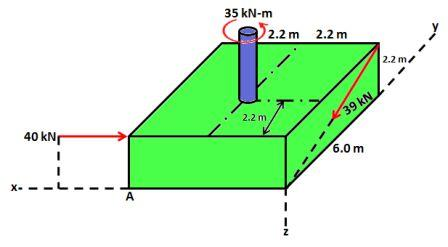# Replace the two forces and a single couple by an equivalent force-couple system at point A.

## Question:

Replace the two forces and a single couple by an equivalent force-couple system at point A.## Equivalent Force-Couple System :

This system comprises of a single force and a moment at a particular desired location.

It produces the same effect at the point as by the various forces and moments producing simultaneously at the same point.

## Answer and Explanation:

Become a Study.com member to unlock this answer! Create your account

Equivalent force at A :

{eq}\vec{F_{A}}=-40\hat{i}-39\times \frac{6}{\sqrt{6^{2}+2.2^{2}}}\hat{j}+39\times \frac{2.2}{\sqrt{6^{2}+2.2^{2}}}\hat{k}...

See full answer below.

#### Learn more about this topic:External Force: Definition & Examples

from

Chapter 1 / Lesson 22
32K

In this lesson, we are going to learn about external forces and their effects on the body to which they are applied. We are also going to distinguish external forces from internal forces.